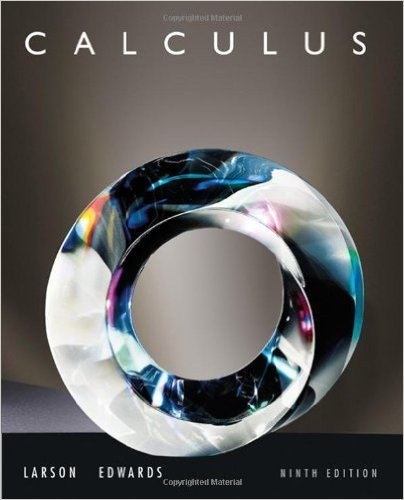×
×

# Solutions for Chapter 7.6: Moments, Centers of Mass, and Centroids## Full solutions for Calculus | 9th Edition

ISBN: 9780547167022Solutions for Chapter 7.6: Moments, Centers of Mass, and Centroids

Solutions for Chapter 7.6
4 5 0 329 Reviews
16
2
##### ISBN: 9780547167022

This expansive textbook survival guide covers the following chapters and their solutions. Since 62 problems in chapter 7.6: Moments, Centers of Mass, and Centroids have been answered, more than 68030 students have viewed full step-by-step solutions from this chapter. Chapter 7.6: Moments, Centers of Mass, and Centroids includes 62 full step-by-step solutions. Calculus was written by and is associated to the ISBN: 9780547167022. This textbook survival guide was created for the textbook: Calculus , edition: 9.

Key Calculus Terms and definitions covered in this textbook
• Arccosecant function

See Inverse cosecant function.

• Axis of symmetry

See Line of symmetry.

• Coefficient

The real number multiplied by the variable(s) in a polynomial term

• Conditional probability

The probability of an event A given that an event B has already occurred

• Continuous at x = a

lim x:a x a ƒ(x) = ƒ(a)

• Dependent event

An event whose probability depends on another event already occurring

• Expanded form of a series

A series written explicitly as a sum of terms (not in summation notation).

• Logarithmic form

An equation written with logarithms instead of exponents

• Natural logarithmic regression

A procedure for fitting a logarithmic curve to a set of data.

• nth root of a complex number z

A complex number v such that vn = z

• Parametrization

A set of parametric equations for a curve.

• Polar coordinate system

A coordinate system whose ordered pair is based on the directed distance from a central point (the pole) and the angle measured from a ray from the pole (the polar axis)

• Positive association

A relationship between two variables in which higher values of one variable are generally associated with higher values of the other variable, p. 717.

• Pythagorean identities

sin2 u + cos2 u = 1, 1 + tan2 u = sec2 u, and 1 + cot2 u = csc2 u

• Richter scale

A logarithmic scale used in measuring the intensity of an earthquake.

• Standard form of a polar equation of a conic

r = ke 1 e cos ? or r = ke 1 e sin ? ,

• Term of a polynomial (function)

An expression of the form anxn in a polynomial (function).

• Terminal point

See Arrow.

• Weighted mean

A mean calculated in such a way that some elements of the data set have higher weights (that is, are counted more strongly in determining the mean) than others.

• Zero of a function

A value in the domain of a function that makes the function value zero.

×